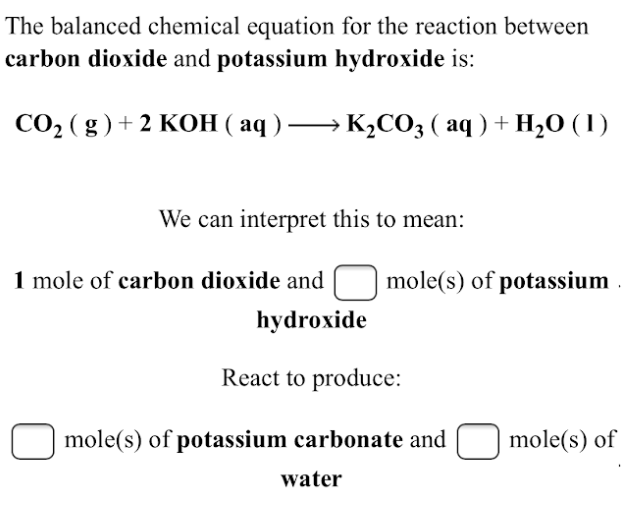# The balanced chemical equation for the reaction between carbon dioxide and potassium hydroxide is: CO2(g) + 2KOH(aq) → K2CO3(aq) + H2O(l) We can interpret this to mean: 1 mole of carbon dioxide and ____ mole(s) of potassium hydroxide. React to produce: ____ moles(s) of potassium carbonate and ____ mole(s) of water